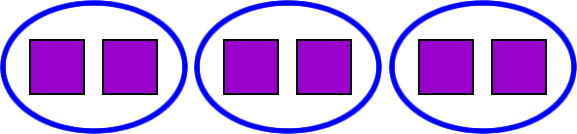Let's see what's really going on when we divide one number into another number.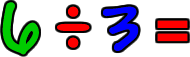We start with 6 triangles: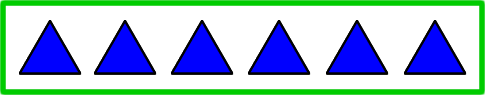We want to see how many chunks of 3 we can make out of 6 things! We can divide 6 things into 2 chunks of 3.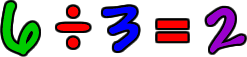Now, let's see how many times 2 will go into 6.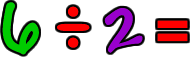Start with 6 squares: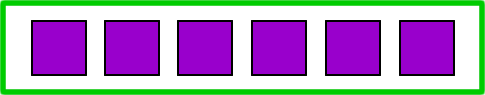We want to see how many chunks of 2 we can make out of 6 things! We can divide 6 things into 3 chunks of 2.